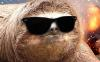Everyone who has never used our Bots before, can test each one for 2 days without any limitation.
The trial is given automatically when you login on the Bot, but in some cases it wouldn't work (security reasons).
If this happens, send me a private message and i will be checking the failed trials manually and adding it for those who didn't get it.
We are looking for resellers who may accept payment methods different from ours, including classictibia's cash, realesta's cash, mastercores' cash, etc. Interested? Click here at anytime.

 FREE Check if character is inside location rangeArkilysAdministratorPosts: 2,532 Threads: 422 Joined: Jul 2018 Reputation: 68 09-17-2021, 04:35 PM Check if character is inside location range (COPY)```local LOCATIONS = {     { X = 32956, RangeX = 2, Y = 32075, RangeY = 2 } -- This will check if you are on X = 32956, 32957 or 32958 AND Y = 32075, 32076 or 32077.     { X = 32956, RangeX = 0, Y = 32075, RangeY = 0 } -- If you wanna check for a specific SQM use RangeX and RangeY = 0. } if standtime() >= 5000 then     local player_x = posx()     local player_y = posy()     local player_z = posz()     for _, temple_location in ipairs(LOCATIONS) do         if (player_x >= temple_location.X and player_x <= (temple_location.X + temple_location.RangeX)) and (player_y >= temple_location.Y and player_y <= (temple_location.Y + temple_location.RangeY)) and player_z == temple_location.Z then             -- do something             break         end     end end``` (COPY)```local LOCATIONS = {     { X = 32957, Y = 32076, Z = 7 },     { X = 32958, Y = 32076, Z = 7 },     { X = 32956, Y = 32076, Z = 7 },     { X = 32957, Y = 32075, Z = 7 },     { X = 32957, Y = 32077, Z = 7 },     { X = 32956, Y = 32075, Z = 7 },     { X = 32958, Y = 32077, Z = 7 },     { X = 32958, Y = 32075, Z = 7 },     { X = 32956, Y = 32077, Z = 7 }, --venore temple } if standtime() >= 5000 then     local player_x = posx()     local player_y = posy()     local player_z = posz()     for _, temple_location in ipairs(LOCATIONS) do         if player_x == temple_location.X and player_y == temple_location.Y and player_z == temple_location.Z then             -- do something             break         end     end end``` keywords: check location, check position, location range, inside location range